# GSEB Solutions Class 7 Maths Chapter 3 Data Handling Ex 3.3

Gujarat Board GSEB Textbook Solutions Class 7 Maths Chapter 3 Data Handling Ex 3.3 Textbook Questions and Answers.

## Gujarat Board Textbook Solutions Class 7 Maths Chapter 3 Data Handling Ex 3.3Question 1.
Use the following bar graph to answer the following questions: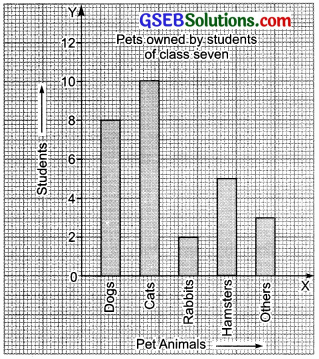(a) Which is the most popular pet?
(b) How may children have dog as a pet.
Solution:
(a) Since the bar representing cats is the highest.
∴ Cat is the most popular pet.
(b) 8 students have dog as a pet.

Question 2.
Read the following bar graph which shows the number of books sold by a booksstore during five consecutive years and answer the following questions:
(i) About how many books were sold in 1989? 1990? 1992?
(ii) In which year were about 475 books soldiAbout 225 books soldi?
(iii) In which years were fewer than 250 books soldi?
(iv) Can you explain how you would estimate the number of books sold in 1989?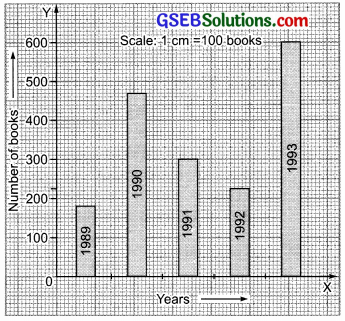Solution:
(i) Number of books sold:

(ii) About 475 books were sold in 1990 and about 225 books were sold in 1992.

(iii) Fewer than 250 books were sold in 1989 and 1992.

(iv) The height of the bar for 1989 is slightly less than that for 200 books. Therefore, about 180 books were sold in 1989.Question 3.
Number of children in six different classes are given below. Represent the data on the bat-graph.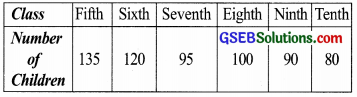(a) How would you choose a scale?
(i) Which class has the maximum number of children? And the minimum?
(ii) Find the ratio of students of class sixth to the students of class eight.
Solution:
(a) Here the greatest value in the data is 135 which is less than 140. Therefore we can choose the scale from 0 to 140 which is neither too long nor too small. By choosing a scale: 1 unit = 10 students and taking class along x-axis, we draw the bar graph as shown: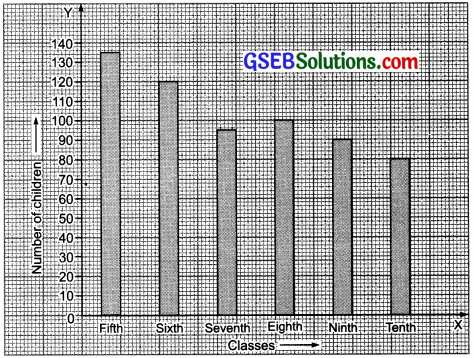(b) (i) Fifth class is having the maximum number of children, i.e. 135 children. Tenth class is having the minimum number of children, i.e. 80 children

(ii) ∵ Number of children in sixth class = 120
Number of children in eighth class =100
∴ The required ratio = $$\frac { 120 }{ 100 }$$
= $$\frac { 6 }{ 5 }$$
= 6 : 5

Question 4.
The performance of a student in 1st Term and 2nd Term is given. Draw a double bar graph choosing appropriate scale and answer the following: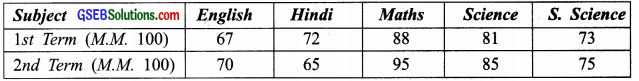(i) In which subject, has the child improved his performance the most?
(ii) In which subject is the improvement the least2
(iii) Has the performance gone down in any subject?
Solution:
The required double bar graph is given below: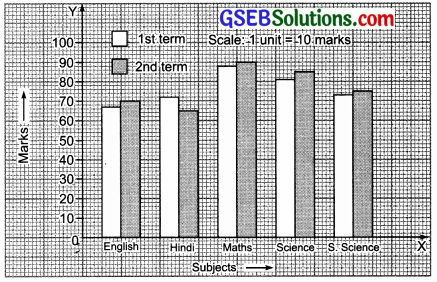(i) Increase in marks
In English = 70 – 67 = 3
In Hindi = 65 – 72 = – 7
In Maths = 95 – 88 = 7
In Science = 85 – 81 = 4
In Social Science = 75 – 73 = 2
Obviously the performance has improved the most in Maths.

(ii) The performance in Social Science is the least.

(iii) Yes, the performance in Hindi has gone down.Question 5.
Consider this data collected from a survey of a colony.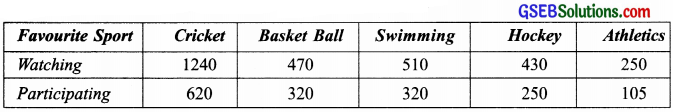(i) Draw a double bar graph choosing an appropriate scale. What do you infer from the bar graphl
(ii) Which sport is most popular?
(iii) Which is more preferred, watching or participating in sports?
Solution:
(i) The required ‘double bar graph’ is given below: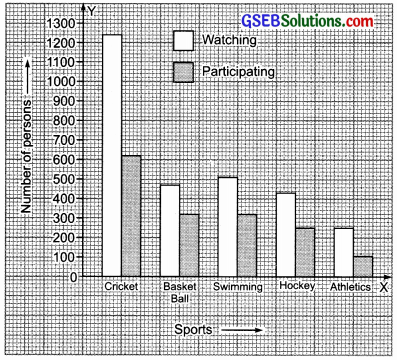From the bar graph it is inferred that the people of the colony like the cricket the most and athletics the least.

(ii) The most popular sport is cricket.

(iii) Watching sports is more preferred than participating in sports.Question 6.
Take the data giving the minimum and the maximum temperature of various cities given in the beginning of this chapter (Table 3.1) Plot a double bar graph using the data and answer the following:
(i) Which city has the largest difference in the minimum and maximum temperature on the given date?
(ii) Which is the hottest city and which is the coldest city?
(iii) Name two cities where maximum temperature of one was less than the minimum temperature of the other.
(iv) Name the city which has the least difference between its minimum and the maximum temperature.
Table 3.1, Page 57 NCERT Textbook
Temperature of cities as on 20.06.2006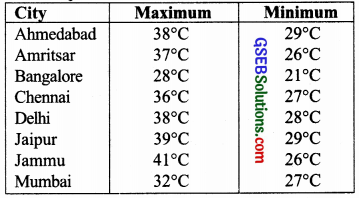Solution:
Plotting a double bar graph, we have: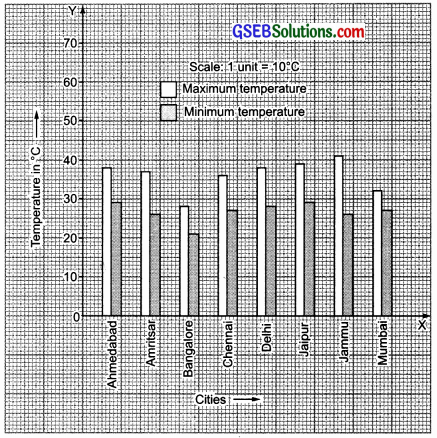From the double bar graph, we have: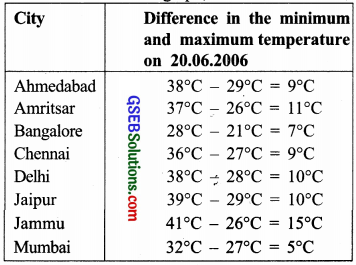(i) Obviously Jammu has the largest difference in the minimum and maximum temperature on the given date.
(ii) Jammu is the hottest city and Bangalore is the coldest city.
(iii) Bangalore and Ahmedabad (or Jaipur).
(iv) Mumbai has the least difference between its maximum temperature and minimum temperature.